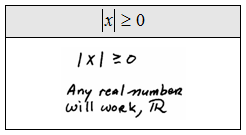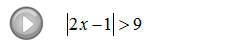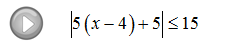## Pages

### Absolute Value Inequalities

Absolute value inequalities and equations are a bit tricky to work with.

There are basically three cases or situations that can arise when working with them.  By guessing and checking we can answer the following three questions.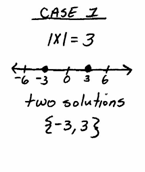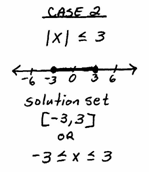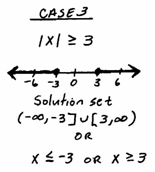Tip: We can easily generalize the above result so that we can use this idea as a template when solving equations and inequalities with absolute values in them. ( Assume n > 0 )

Case 1: | | = n can be solved using X = -n or X = n.
Case 2: | | < n can be solved using -n < X < n.
Case 3: | | > n can be solved using X < -n or X > n.

Use the following steps to solve an absolute value equation or inequality.

Step 1: Isolate the absolute value.
Step 2: Identify the case and apply the appropriate theorem.
Step 3: Solve the resulting equation or inequality.
Step 4: Graph the solution set and express it in interval notation.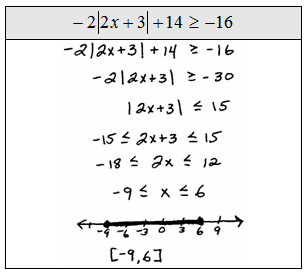Instructional Video: Absolute Value Inequalities

Solve and graph the solution set.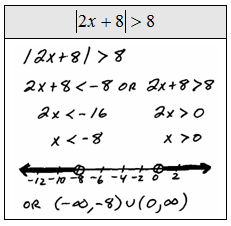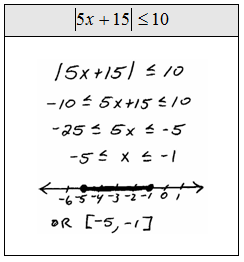In the three cases listed above notice that we took care to say that n > 0.  The next three problems illustrate some of the situations encountered when n = 0. Plug in some numbers and see what happens.